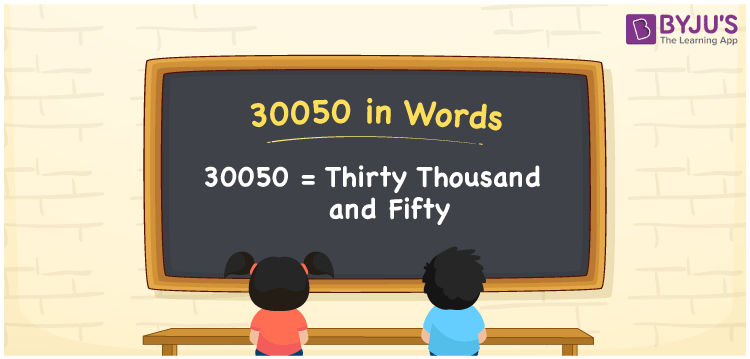# 30050 in Words

30050 in words is “Thirty thousand and fifty”. For example, Akshaya has spent Rs. 30050 for purchasing books. It can also be written as “Akshaya has spent Rs. Thirty thousand and fifty for purchasing books”. Now, let us learn in detail about the procedure of writing the number 30050 using the place value system.

 30050 in Words: Thirty Thousand and Fifty. Thirty Thousand and Fifty in Numerical Form: 30050.

## 30050 in English Words## How to Write 30050 in Words?

The following table presents the place values of the number 30050.

 Ten-thousands Thousands Hundreds Tens Ones 3 0 0 5 0

The expanded form of 30050 is as follows:

= 3 × Ten thousand + 0 × Thousand + 0 × Hundred + 5 × Ten + 0 × One

= 3 × 10000 + 0 × 1000 + 0 × 100 + 5 × 10 + 0 × 1

= 30000 + 50

= 30050

= Thirty thousand and fifty

Hence, 30050 in words is thirty thousand and fifty.

30050 in words – Thirty thousand and fifty

Is 30050 an odd number? – No

Is 30050 an even number? – Yes

Is 30050 a perfect square number? – No

Is 30050 a perfect cube number? – No

Is 30050 a prime number? – No

Is 30050 a composite number? – Yes

## Frequently Asked Questions on 30050 in Words

Q1

### How to spell 30050?

30050 in words is thirty thousand and fifty.

Q2

### Simplify 30000 + 50, and express it in words.

Simplifying 30000 + 50, we get 30050. Hence, 30050 in words is thirty thousand and fifty.

Q3

### Is 30050 an odd number?

No, 30050 is not an odd number.